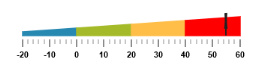# Ranges

21 Apr 20176 minutes to read

Ranges are used to specify or group the scale values. You can describe the values in the pointers using ranges.

Range collection can be directly added to the scale object. Refer the following code example to add range collection in a Linear Gauge control.

• CSHTML
• ``````<ej-linear-gauge id="LinearGauge" enable-animation="false" height="150" width="600"
orientation="@Orientation.Horizontal" label-color="black" is-responsive="true" >
<e-linear-scale-collections>
<e-linear-scales width="0" maximum="60" minimum="-20" directions="@Directions.Clockwise" background-color="transparent"
show-marker-pointers="true" show-bar-pointers="false" show-custom-labels="true"
show-ranges="true">
<e-border color="transparent" width="1"></e-border>
<e-linear-label-collections>
<e-linear-labels >
<e-distance-from-scale x="0" y="50" ></e-distance-from-scale>
</e-linear-labels>
</e-linear-label-collections>
<e-linear-tick-collections>
<e-linear-ticks type="@TickType.MajorInterval"  width="2" color="#8c8c8c">
<e-distance-from-scale x="25" y="0"></e-distance-from-scale>
</e-linear-ticks>
<e-linear-ticks type="@TickType.MinorInterval"  width="1" color="#8c8c8c" >
<e-distance-from-scale x="25" y="0"></e-distance-from-scale>
</e-linear-ticks>
</e-linear-tick-collections>
<e-marker-pointer-collections>
<e-marker-pointers width="3" length="30" markerdistance-from-scale="20" type="@MarkerType.Star"
marker-background-color="#FE5C09" placement="@PointerPlacement.Near" value="55">
</e-marker-pointers>
</e-marker-pointer-collections>
<e-linear-range-collections>
<e-linear-ranges end-value="60" start-value="-20" placement="@RangePlacement.Near" distance-from-scale="20" range-background-color="#FEBE48" start-width="0" end-width="20">
</e-linear-ranges>
</e-linear-range-collections>
</e-linear-scales>
</e-linear-scale-collections>
</ej-linear-gauge>``````

Execute the above code to render the following output.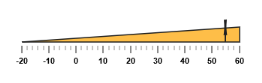## Range Customization

### Appearance

The major attributes for ranges are startValue and endValue. The startValue defines the start position of the range and endValue defines the end position of the range. The startWidth and endWidth are used to specify the range width at the starting and ending position of the ranges.

• CSHTML
• ``````<ej-linear-gauge id="LinearGauge" enable-animation="false"  width="500"
label-color="#8c8c8c" >
<e-linear-scale-collections>
show-marker-pointers="false" show-bar-pointers="false"
show-ranges="true">
<e-border color="transparent" width="1"></e-border>
<e-linear-label-collections>
<e-linear-labels >
<e-font size="11px" font-family="Segoe UI" font-style="bold"></e-font>
</e-linear-labels>
</e-linear-label-collections>
<e-linear-tick-collections>
<e-linear-ticks type="@TickType.MajorInterval"  width="1" color="#8c8c8c">
</e-linear-ticks>
</e-linear-tick-collections>
<e-linear-range-collections>
<e-linear-ranges end-value="50" start-value="0" distance-from-scale="5"
range-background-color="#F6B53F" start-width="8" end-width="8">
</e-linear-ranges>
<e-linear-ranges end-value="100" start-value="70" distance-from-scale="5"
range-background-color="#E94649" start-width="8" end-width="8">
</e-linear-ranges>
</e-linear-range-collections>
</e-linear-scales>
</e-linear-scale-collections>
</ej-linear-gauge>``````

Execute the above code to render the following output.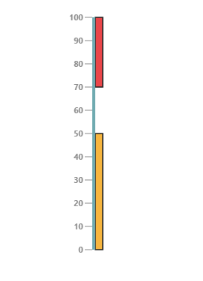### Colors and Border

• You can customize the ranges to improve the appearance of the Gauge. The range border is modified with the object called border. It has two border property such as color and width which are used to customize the border color of the ranges and border width of the ranges.
• You can set the background color to improve the look and feel of the Linear Gauge. For customizing the background color of the ranges, backgroundColor is used.You can add the gradient effects to the ranges by using gradient object.
• CSHTML
• ``````<ej-linear-gauge id="LinearGauge"  width="500"
label-color="#8c8c8c" >
<e-linear-scale-collections>
<e-linear-scales width="4" background-color="transparent" length="310"
show-marker-pointers="false" show-bar-pointers="false"
show-ranges="true">
<e-border color="transparent" width="1"></e-border>
<e-linear-label-collections>
<e-linear-labels >
<e-font size="11px" font-family="Segoe UI" font-style="bold"></e-font>
</e-linear-labels>
</e-linear-label-collections>
<e-linear-tick-collections>
<e-linear-ticks type="@TickType.MajorInterval"  width="1" color="#8c8c8c">
</e-linear-ticks>
</e-linear-tick-collections>
<e-linear-range-collections>
<e-linear-ranges end-value="50" start-value="0" distance-from-scale="5"
range-background-color="#F6B53F" start-width="18" end-width="3">
<e-border color="black" width="2"></e-border>
</e-linear-ranges>
<e-linear-ranges end-value="100" start-value="70" distance-from-scale="10"
range-background-color="#E94649" start-width="18" end-width="8">
<e-border color="black" width="2"></e-border>
</e-linear-ranges>
</e-linear-range-collections>
</e-linear-scales>
</e-linear-scale-collections>
</ej-linear-gauge>``````

Execute the above code to render the following output.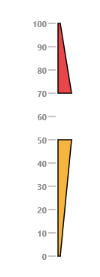## Positioning the ranges

• You can position ranges using two properties such as distanceFromScale and placement. The distanceFromScale property defines the distance between the scale and range.
• Placement property is used to locate the pointer with respect to scale either inside the scale or outside the scale or along the scale. It is an enumerable data type.
• CSHTML
• ``````<ej-linear-gauge id="LinearGauge"  width="500"
label-color="#8c8c8c">
<e-linear-scale-collections>
<e-linear-scales width="4" background-color="transparent" length="310"
show-marker-pointers="false" show-bar-pointers="false"
show-ranges="true">
<e-border color="transparent" width="1"></e-border>
<e-linear-label-collections>
<e-linear-labels >
<e-font size="11px" font-family="Segoe UI" font-style="bold"></e-font>
</e-linear-labels>
</e-linear-label-collections>
<e-linear-tick-collections>
<e-linear-ticks type="@TickType.MajorInterval"  width="1" color="#8c8c8c">
</e-linear-ticks>
</e-linear-tick-collections>
<e-linear-range-collections>
<e-linear-ranges end-value="50" start-value="0" distance-from-scale="-30"
range-background-color="#F6B53F" start-width="18" end-width="3" placement="@RangePlacement.Near">
<e-border color="black" width="2"></e-border>
</e-linear-ranges>
<e-linear-ranges end-value="100" start-value="70" distance-from-scale="-30"
range-background-color="#E94649" start-width="18" end-width="8" placement="@RangePlacement.Near">
<e-border color="black" width="2"></e-border>
</e-linear-ranges>
</e-linear-range-collections>
</e-linear-scales>
</e-linear-scale-collections>
</ej-linear-gauge>``````

Execute the above code to render the following output.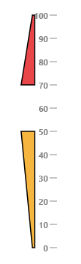## Multiple Ranges

You can set multiple ranges by adding an array of range objects. Refer the following code example for multiple range functionality.

• CSHTML
• ``````<ej-linear-gauge id="LinearGauge"  width="600" height="150"  orientation="@Orientation.Horizontal"
label-color="Black" is-responsive="true">
<e-linear-scale-collections>
<e-linear-scales width="0" background-color="transparent" length="310"
show-marker-pointers="true" show-bar-pointers="false" show-custom-labels="true" direction="@Directions.Clockwise"
minimum="-20" maximum="60" show-ranges="true">
<e-border color="transparent" width="1"></e-border>
<e-linear-label-collections>
<e-linear-labels >
<e-distance-from-scale x="0" y="50" ></e-distance-from-scale>
</e-linear-labels>
</e-linear-label-collections>
<e-linear-tick-collections>
<e-linear-ticks type="@TickType.MajorInterval"  width="2" color="#8c8c8c">
<e-distance-from-scale x="-1" y="20" ></e-distance-from-scale>
</e-linear-ticks>
<e-linear-ticks type="@TickType.MinorInterval"  width="1" height="6" color="#8c8c8c">
<e-distance-from-scale x="-1" y="20" ></e-distance-from-scale>
</e-linear-ticks>
</e-linear-tick-collections>
<e-marker-pointer-collections>
<e-marker-pointers width="30" length="30" markerdistance-from-scale="20" type="@MarkerType.Star"
marker-background-color="#FE5C09" placement="@PointerPlacement.Near" value="55">
</e-marker-pointers>
</e-marker-pointer-collections>
<e-linear-range-collections>
<e-linear-ranges end-value="0" start-value="-20" distance-from-scale="20"
range-background-color="#2788B1" start-width="5" end-width="10" placement="@RangePlacement.Near">
<e-border color="#2788B1" width="2"></e-border>
</e-linear-ranges>
<e-linear-ranges end-value="20" start-value="0" distance-from-scale="20"
range-background-color="#A5BA28" start-width="10" end-width="15" placement="@RangePlacement.Near">
<e-border color="#A5BA28" width="2"></e-border>
</e-linear-ranges>
<e-linear-ranges end-value="40" start-value="20" distance-from-scale="20"
range-background-color="#FEBE48" start-width="15" end-width="20" placement="@RangePlacement.Near">
<e-border color="#FEBE48" width="2"></e-border>
</e-linear-ranges>
<e-linear-ranges end-value="60" start-value="40" distance-from-scale="20"
range-background-color="Red" start-width="20" end-width="25" placement="@RangePlacement.Near">
<e-border color="Red" width="2"></e-border>
</e-linear-ranges>
</e-linear-range-collections>
</e-linear-scales>
</e-linear-scale-collections>
</ej-linear-gauge>``````

Execute the above code to render the following output.